## ML Aggarwal Class 6 Solutions for ICSE Maths Chapter 11 Understanding Symmetrical Shapes Ex 11.2

Question 1.
What fraction of a clockwise revolution does the hour hand of a clock turn through when it goes from:
(i) 4 to 10
(ii) 2 to 5
(iii) 7 to 10
(iv) 8 to 5
(v) 11 to 5
(vi) 6 to 3
Also find the number of right angles turned in each case.
Solution: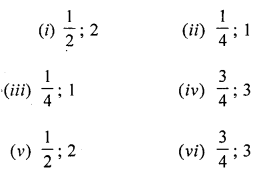Question 2.
Where will the hand of a clock stop if it
(i) starts at 10 and makes $$\frac{1}{2}$$ of a revolution, clockwise?
(ii) starts at 4 and makes $$\frac{1}{4}$$ of a revolution, clockwise?
(iii) starts at 4 and makes $$\frac{3}{4}$$ of a revolution, clockwise?
Solution:
(i) at 4
(ii) at 7
(iii) at 1

Question 3.
Where will the hand of a clock stop if it starts from
(i) 6 and turns through 1 right angle?
(ii) 8 and turns through 2 right angles?
(iii) 10 and turns through 3 right angles?
(iv) 7 and turns through 2 straight angles?
Solution:
(i) 9
(ii) 2
(iii) 1
(iv) 7

Question 4.
What fraction of a revolution have you turned through if you stand facing
(i) north and turn clockwise to face west?
(ii)south and turn anti-clockwise to face east?
(iii) east and turn clockwise (or anti-clockwise) to face west?
Also find the number of right angles turned in each case.
Solution:Question 5.
Match the followingSolution: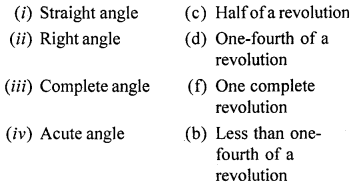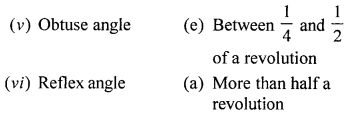Question 6.
Classify the angles whose magnitudes are given below:
(i) 56°
(ii) 125°
(iii) 90°
(iv) 180°
(v) 215°
(vi) 328°
(vii) 89°
(viii) 178°
Solution:
(i) Acute angle
(ii) Obtuse angle
(iii) Right angle
(iv) Straight angle
(v) Reflex angle
(vi) Reflex angle
(vii) Acute angle
(viii) Obtuse angle

Question 7.
State which of the following angles with a small letter in the following diagrams are acute, which obtuse and which are reflex: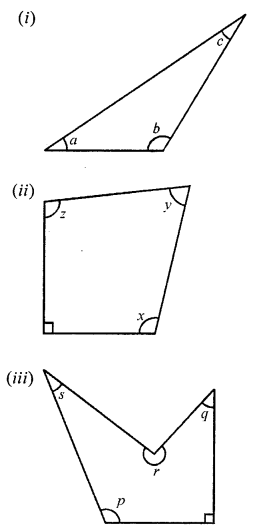Solution:
(i) ∠a and ∠c are acute, ∠h is obtuse
(ii) ∠x and ∠z are obtuse, ∠y is acute.
(iii) ∠p is obtuse, ∠q and ∠s are acute, ∠r is reflex.

Question 8.
Use your protector to measure each of the angles marked in the following figures: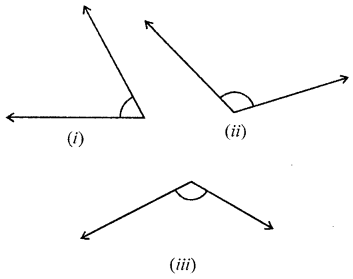Solution:
(i) 62°
(ii) 116°
(iii) 121°

Question 9.
Use your protector to measure the reflex angles marked in the following figures:Solution:
(i) 315°
(ii) 235°

Question 10.
Find the measure of the angle between the hands of the clock in each figure:Solution:
(i) 60°
(ii) 30°
(iii) 150°

Question 11.
Write the measure of the smaller angle formed by the hour and the minutes hands of a clock at 7 O’clock. Also write the measure of the other angle and also state what types of angles these are.
Solution:
Smaller angle is 150° (obtuse).
The other angle is 210° (reflex).

Question 12.
There are two set-squares in your geometry box. What are measures of the angles formed at their corners? Do they have any angle measure that is common?
Solution:
One is a 30° – 60° – 90° set square; the other is 45° – 45° – 90° set square. The angle of measure 90° (i.e., a right angle) is common between them.